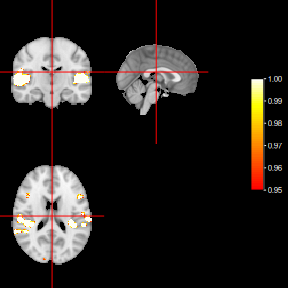# Individual_analysis

library(BayesDLMfMRI)
library(oro.nifti)
library(neurobase)

To run any of the functions related to individual analysis, the user must provide two inputs: an array of four dimensions containing the sequence of MRI images and a matrix whose columns have the covariates to model the observed BOLD response. Thus, we load the data, which contains the MRI images, using the function. The covariates, specifically, the expected BOLD response and its derivative, are contained in the covariates dataframe The rationale for including the temporal derivative is that this basis can capture small offsets in the time to peak of the hemodynamic response

# load example data
fMRI.data <- get_example_fMRI_data()
fMRI.data  |> dim()
#>   91 109  91 310
# load example covaraites
data("covariates", package="BayesDLMfMRI")

Covariates |> dim()
#>  310   2

To perform an individual voxel-wise analysis and consequently obtain a 3D array of measurements of activation, the user can choose among three different functions: ffdEvidenceFETS, ffdEvidenceFFBS and ffdEvidenceFSTS. These functions can yield three types of evidence measurements for voxel activation: Marginal effect, Average cluster effect, and Joint effect. To illustrate their use and functionality, we run an example from the “voice localizer” data.

res <- ffdEvidenceFETS(ffdc = fMRI.data,
covariates = Covariates,
m0 = 0, Cova = 100, delta = 0.95,
S0 = 1, n0 = 1, Nsimu1 = 100, Cutpos1 = 30,
r1 = 1, Test = "LTT", Ncores = 15)


The arguments m0, Cova, S0 and n0 are the hyper-parameters related to the joint prior distribution of $$(\Theta_{{[i,j,k]t\mathstrut}}^{{(z)\mathstrut}}, \Sigma_{{[i,j,k]t\mathstrut}}^{{(z)\mathstrut}})$$. For this example, we are setting a “vague” prior distribution according to Quintana and West , where m0 = 0 define a null matrix with zero values in all its entries. And both Cova = 100 and S0 = 1 define respectively the values for the diagonal matrices $$C_0$$ and $$S_0$$ as defined in Cardona-Jiménez and Pereira . r1 is the euclidean distance, which defines the size of the cluster of voxels jointly modeled. Test is the parameter related to the test selected by the user, for which there are two options: “LTT” and “Joint”. “Ncores” is the argument related to the number of cores when the process is executed in parallel. Nsimu1 is the number of simulated on-line trajectories related to the state parameter $$\Theta_{{[i,j,k]t\mathstrut}}^{{(z)\mathstrut}}$$. From our own experience dealing with different sets of fMRI data, we recommend Nsimu1 = 100 as a good number of draws to obtain reliable results. Cutpos1 is the time up from where the on-line trajectories are considered in the computation of the activation evidence, and delta is the value of the discount factor. For a better understanding about the setting of these two last arguments, see Cardona-Jiménez and Pereira .

str(res)
#> List of 2
#>  $: num [1:91, 1:109, 1:91] 0 0 0 0 0 0 0 0 0 0 ... #>$ : num [1:91, 1:109, 1:91] 0 0 0 0 0 0 0 0 0 0 ...
#>  - attr(*, "class")= chr "fMRI_single_evidence"
dim(res[])
#>   91 109  91

The output for the ffdEvidenceFEST function depends on the type of Test set by the user. For Test = “LTT” the function returns a list of the type res[[p]][x, y, z], where p represents the column position in the covariates matrix and [x, y, z] represent the voxel position in the brain image. Thus, for the “voice localizer” example res[] and res[] are the 3D arrays related to the evidence for brain activation associated to the BOLD response for the auditory stimuli and its derivative respectively. When Test = Joint the output returned is an array of the type res[[2*p]][x, y, z], with the first p elements of the array related to the Joint effect distribution and the rest of it to the Marginal effect distribution.

plot(res, overlay=ffd, index=1, col.y = heat.colors(50), ycolorbar = TRUE, ybreaks = seq(0.95, 1, by = 0.001))The neurobase package is one option available in R to visualize MRI images. In this example, we use its ortho2() function to plot the evidence activation map. The ffd data contains the MNI brain atlas, which is used in this example as a reference space for individual and group analysis. For a better understanding of the use of brain atlas, see Brett et al. .